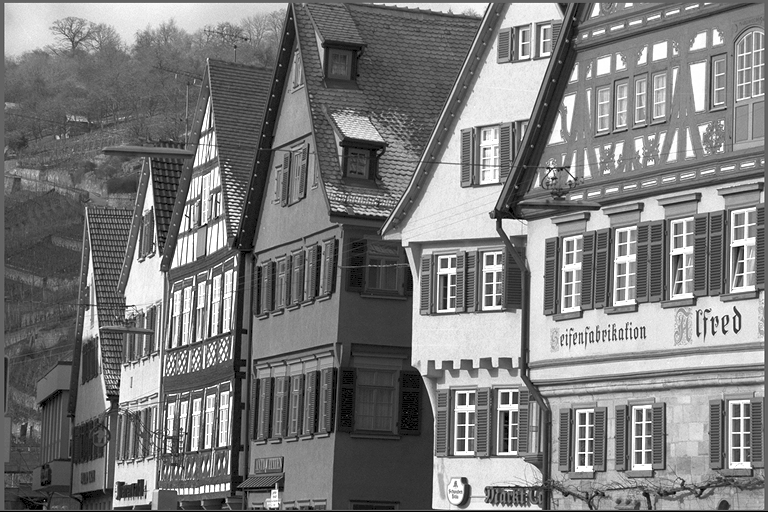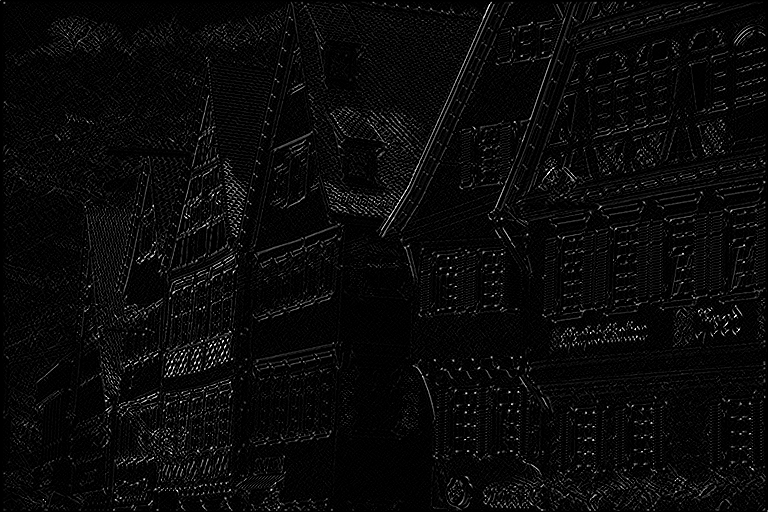Convolution

# Overview

The Convolution algorithm performs a 2D convolution operation on the input image with the provided 2D kernel. This is useful when the kernel isn't separable and its dimensions are smaller than 5x5. In other cases, it's usually preferable to use the Separable Convolution algorithm due to its speed.

Input Kernel Output$\begin{bmatrix} 1 & 0 & -1 \\ 0 & 0 & 0 \\ -1 & 0 & 1 \end{bmatrix}$# Implementation

Discrete 2D convolution is implemented using the following discrete function:

$I'[x,y] = \sum_{m=0}^{k_h} \sum_{n=0}^{k_w} K[m,n] \times I[x-(n-\lfloor k_w/2 \rfloor), y-(m-\lfloor k_h/2 \rfloor) ]$

Where:

• $$I$$ is the input image.
• $$I'$$ is the result image.
• $$K$$ is the convolution kernel.
• $$k_w,k_h$$ are the kernel's width and height, respectively.
Note
Most computer vision libraries expect the kernel to be reversed before calling their convolution functions. Not so with VPI, we implement an actual convolution, not cross-correlation. Naturally, this is irrelevant if the kernel is symmetric.

# C API functions

For list of limitations, constraints and backends that implements the algorithm, consult reference documentation of the following functions:

Function Description
vpiSubmitConvolution Runs a generic 2D convolution over an image.

# Usage

Language:
1. Import VPI module
import vpi
2. Define a 3x3 convolution kernel to perform edge detection.
kernel = [[ 1, 0, -1],
[ 0, 0, 0],
[-1, 0, 1]]
3. Run convolution filter on input image using the CPU backend and the given kernel. Input and output are VPI images.
with vpi.Backend.CUDA:
output = input.convolution(kernel, border=vpi.Border.ZERO)
1. Initialization phase
1. Include the header that defines the needed functions and structures.
Declares functions to perform image filtering with convolution kernels.
2. Define the input image object.
VPIImage input = /*...*/;
struct VPIImageImpl * VPIImage
A handle to an image.
Definition: Types.h:256
3. Create the output image. It gets its dimensions and format from the input image.
int32_t w, h;
vpiImageGetSize(input, &w, &h);
vpiImageGetFormat(input, &type);
VPIImage output;
vpiImageCreate(w, h, type, 0, &output);
uint64_t VPIImageFormat
Pre-defined image formats.
Definition: ImageFormat.h:94
VPIStatus vpiImageGetFormat(VPIImage img, VPIImageFormat *format)
Get the image format.
VPIStatus vpiImageCreate(int32_t width, int32_t height, VPIImageFormat fmt, uint64_t flags, VPIImage *img)
Create an empty image instance with the specified flags.
VPIStatus vpiImageGetSize(VPIImage img, int32_t *width, int32_t *height)
Get the image dimensions in pixels.
4. Create the stream where the algorithm will be submitted for execution.
VPIStream stream;
vpiStreamCreate(0, &stream);
struct VPIStreamImpl * VPIStream
A handle to a stream.
Definition: Types.h:250
VPIStatus vpiStreamCreate(uint64_t flags, VPIStream *stream)
Create a stream instance.
2. Processing phase
1. Define the kernel to be used. In this case, a simple 3x3 edge detector.
float kernel[3 * 3] = { 1, 0,-1,
0, 0, 0,
-1, 0, 1};
2. Submit the algorithm to the stream, passing the kernel and other parameters. It'll be executed by the CPU backend.
vpiSubmitConvolution(stream, VPI_BACKEND_CPU, input, output, kernel, 3, 3, VPI_BORDER_ZERO);
VPIStatus vpiSubmitConvolution(VPIStream stream, uint64_t backend, VPIImage input, VPIImage output, const float *kernelData, int32_t kernelWidth, int32_t kernelHeight, VPIBorderExtension border)
Runs a generic 2D convolution over an image.
@ VPI_BACKEND_CPU
CPU backend.
Definition: Types.h:92
@ VPI_BORDER_ZERO
All pixels outside the image are considered to be zero.
Definition: Types.h:278
3. Optionally, wait until the processing is done.
vpiStreamSync(stream);
VPIStatus vpiStreamSync(VPIStream stream)
Blocks the calling thread until all submitted commands in this stream queue are done (queue is empty)...
3. Cleanup phase
1. Free resources held by the stream and the input and output images.
vpiImageDestroy(output);
void vpiImageDestroy(VPIImage img)
Destroy an image instance.
void vpiStreamDestroy(VPIStream stream)
Destroy a stream instance and deallocate all HW resources.

Consult the Image Convolution for a complete example.

For more information, see Convolution in the "C API Reference" section of VPI - Vision Programming Interface.

# Performance

For information on how to use the performance table below, see Algorithm Performance Tables.
Before comparing measurements, consult Comparing Algorithm Elapsed Times.
For further information on how performance was benchmarked, see Performance Benchmark.

-